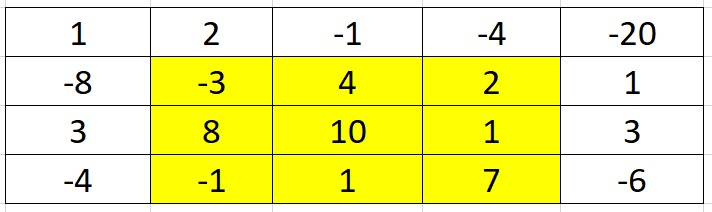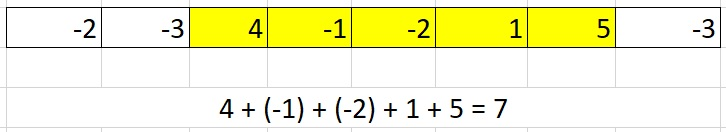×

Search anything:

# Maximum Sum Rectangle in a Matrix

#### Algorithms Dynamic Programming (DP)Reading time: 35 minutes | Coding time: 10 minutes

In this article, the problem we are going to discuss is - given a 2D array, we need to find the subarray with the maximum sum of its elements. As negative numbers can be present within the 2D array, this problem is challenging.

We solve this in two approaches:

• Brute force solution O(N^5)
• Dynamic Programming solution O(N^3)

Example:From amongst all rectangles possible in the above matrix, the one marked across with the blue outline depicts the maximum sum rectangle(in this case, a square). All other configurations of rectangles have positive values of sum but the maximum sum for this example is 29.

This problem is an extension of Kadane algorithm for 2D matrices where Kadane's algorithm is for 1D array. Before going into the actual solution, we shall explore the brute force approach.

## Brute Force solution O(N^5)

Check every possible rectangle in the 2D array. With this approach, we need to select 2 row-indexes and 2 column-indexes and hence compute the sum of each possible rectangle. The maximum sum rectangle we obtain would provide us with the answer.

However, since the row and column indexes are variable, the problem requires four loops and with 2 inner loops to calculate the sum, the time-complexity of this apporach is O(N^6)

The time complexity of calculating the sum can be reduced from O(N^2) to O(N) using prefix sum array and hence, the time complexity can be brough down to O(N^5).

## Dynamic Programming solution O(N^3)

This problem is mainly an extension of calculating the maximum sum contiguous subarray, which can easily be implemented using Kadane's algorithm.

Now we just need contiguous sets of rows that form a rectangle of maximum possible sum in the matrix. When values in the matrix are all positive the answer is pretty straight forward, the maximum sum rectangle is the matrix itself.

We use dynamic programming to reduce the brute force time complexity to O(N^3).

The idea is to fix the left and right columns one by one and find the maximum sum contiguous rows for every left and right column pair.

We then find top and bottom row numbers for every fixed left and right column pair, which produces the maximum sum for us.

To find the top and bottom row numbers, calculate sum of elements in every row from left to right and store these sums in an array, say temp[]. Hence, temp[i] indicates the sum of elements from left to right in row 'i'.

If we apply Kadane’s algorithm on temp[], and get the maximum sum subarray of temp, this maximum sum would be the maximum possible sum with left and right as boundary columns. To get the overall maximum sum, we compare this sum with the maximum sum obtained so far.

``````1. initialize max_so_far = 0, max_ending_here = 0
2. loop for each element of the array
a) max_ending_here += a[i]
b) if(max_ending_here<0)
max_ending_here = 0
if(max_so_far<max_ending_here)
max_so_far = max_ending_here
3. return max_so_far
``````

In the above algorithm, two variables with the name max_so_far and ma_endinh_here are initially zero. We keep on adding the elements of array to max_ending_here. If the sum(max_ending_here) <0, meaning negative elements are not helpful in yielding maximum contiguous sum. If max_so_far <max_ending_here, we store it.On encountering negative elements that make max_ending_here negative, max_ending_here is again zero and we further calculate max_ending_here.If again the max_so_far < max_ending_here,
we assign it again. Effectively, Kadane's algorithm calculates sum within negative values occuring in the array and assigns the maximum subarray sum to max_so_far.

``````int kadane(vector<int>&arr)
{
int n = arr.size()
int max_so_far = 0, max_ending_here = 0

for(int i=0; i<n; i++){
max_ending_here = max(max_ending_here,
max_ending_here+arr[i])
if(max_so_far<max_ending_here)
max_so_far = max_ending_here
}

return max_so_far
}
``````Explanation to the example above:-

n = 8 //array size
max_so_far = 0, max_ending_here = 0

fisrt iteration,
i=0, a = -2
max_ending_here = max_ending_here + (-2)
max_ending_here = 0 as max_ending_here < 0

second iteration,
i=1, a = -3
max_ending_here = max_ending_here + (-3)
Set max_ending_here = 0 as max_ending_here < 0

third iteration,
i=2, a = 4
max_ending_here = max_ending_here + (4)
max_ending_here = 4
max_so_far is updated to 4 because max_ending_here greater
than max_so_far which was 0 till now

fourth iteration,
i=3, a = -1
max_ending_here = max_ending_here + (-1)
max_ending_here = 3 // no change here to
// make max_ending_here = 0

fifth iteration,
i=4, a = -2
max_ending_here = max_ending_here + (-2)
max_ending_here = 1

sixth iteration,
i=5, a = 1
max_ending_here = max_ending_here + (1)
max_ending_here = 2

seventh iteration,
i=6, a = 5
max_ending_here = max_ending_here + (5)
max_ending_here = 7
max_so_far is updated to 7 because max_ending_here is
greater than max_so_far

eighth iteration,
i=7, a = -3
max_ending_here = max_ending_here + (-3)
max_ending_here = 4

as (max_so_far = 7) > (max_ending_here = 4),
max_so_far = 7

return 7

since number of iterations = array size,
time complexity of Kadane's Algorithm = O(n) // n, arr size

## Pseudocode to extend Kadane's algorithm

Consider the following pseudocode where is find the rectangle with the maximum sum using Kadane's algorithm as a sub-routine:

``````int findMaxSum(int Matrix[][col])
{
// Variables to store the final output
int maxSum = INT_MIN;
int finalLeft, finalRight, finalTop, finalBottom;
//dimensions

int left, right, i;  //left will iterate till col
int temp[row], sum, start, finish;
// temp[row] is auxiliary space

// Set the left column
for (left = 0; left < col; left++)
{
// Initialize all elements of temp as 0
memset(temp, 0, sizeof(temp));

// Set the right column for the left
// column set by outer loop
for (right = left; right < col; right++)
{

// Calculate sum between current left and right for
every row, i
for (i = 0; i < row; i++)
temp[i] += M[i][right];

// Find the maximum sum subarray in temp[].
// The kadane() function also sets values
// of start and finish. So sum is sum of
// rectangle between (start, left) and
// (finish, right) which is the maximum sum
// with boundary columns strictly as left
// and right.

//call kadane() and store in sum
sum = kadane(temp, &start, &finish, row);

// Compare sum with maximum sum so far.
// If sum is more, then update maxSum and
// other output values to fix rectangle dimensions
if (sum > maxSum)
{
maxSum = sum;
finalLeft = left;
finalRight = right;
finalTop = start;
finalBottom = finish;
}
}
}

return maxSum;
}
``````

## Implementation

Here goes the implementation of the above method:-

``````#include<bits/stdc++.h>
using namespace std;

int row
int col

/* Implementation of Kadane's algorithm for 1D array. This function returns the maximum sum and stores start and end indexes of the maximum sum subarray at addresses stored in the start and finish respectively*/

int* finish, int n)  //n is array size
{
// initialize sum, maxSum and
int sum = 0, maxSum = INT_MIN, i;  //INT_MIN is minimum
// integer value

// for all negative values case
*finish = -1;

// local iterator if sum<0, start is local_start
int local_start = 0;

for (i = 0; i <n; i++)
{
sum += arr[i];
if (sum < 0)
{
sum = 0;
local_start = i + 1;
}
else if (sum > maxSum)
{
maxSum = sum;
*start = local_start;
*finish = i;
}
}

// if atleast one non -ve number
if (*finish != -1)
return maxSum;

// Special Case: When all numbers
// in arr[] are negative
maxSum = arr;
*start = *finish = 0;  //maximum sum = 0

// Find maximum element in array
for (i = 1; i < n; i++)
{
if (arr[i] > maxSum)
{
maxSum = arr[i];
*start = *finish = i;
}
}
return maxSum;
}

//function to find maximum sum rectangle in Matrix[][]
int findMaxSum(int Matrix[][col])
{
// Variables to store the final output
int maxSum = INT_MIN;
int finalLeft, finalRight, finalTop, finalBottom;
//dimensions

int left, right, i;  //left will iterate till col
int temp[row], sum, start, finish;
// temp[row] is auxiliary space

// Set the left column
for (left = 0; left < col; left++)
{
// Initialize all elements of temp as 0
memset(temp, 0, sizeof(temp));

// Set the right column for the left
// column set by outer loop
for (right = left; right < col; right++)
{

// Calculate sum between current left and right for
every row, i
for (i = 0; i < row; i++)
temp[i] += M[i][right];

// Find the maximum sum subarray in temp[].
// The kadane() function also sets values
// of start and finish. So sum is sum of
// rectangle between (start, left) and
// (finish, right) which is the maximum sum
// with boundary columns strictly as left
// and right.

//call kadane() and store in sum
sum = kadane(temp, &start, &finish, row);

// Compare sum with maximum sum so far.
// If sum is more, then update maxSum and
// other output values to fix rectangle dimensions
if (sum > maxSum)
{
maxSum = sum;
finalLeft = left;
finalRight = right;
finalTop = start;
finalBottom = finish;
}
}
}

return maxSum;
}

// main
int main()
{
int Matrix[ROW][COL] = {{1, 2, -1, -4, -20},
{-8, -3, 4, 2, 1},
{3, 8, 10, 1, 3},
{-4, -1, 1, 7, -6}};

cout<<findMaxSum(Matrix);

return 0;
}

``````

## Explanation

For the example above,

left = 0
{
right = 0 to 5{
temp[] = {0,0,0,0},
i=0 to 4, temp[] = {1,-8,-3,-4}
left = 0, right = 0, start = 2, end = 2,
i=0 to 4, temp[] = {3,-11,11,-5}
left = 0, right = 1, start = 2, end = 2,
... till right = 3
}
set temp[] = {0,0,0,0}
continue for left = 1,2,3,4 and set dimensions accordingly,
keep building the temp array and set dimensions
to get sum = 29
}
...till left = 4

## Time Complexity Analysis

2 loops of iterators left and right run from 0 till col,
and the inner loop calls kadane which is of linear complexity
and initialization of temp array is linear;

time = O(n) * O(n) * {O(n) + O(n)}
time = O(n^3).

Thus, the time complexity of the above implementation is O(N^3) which is better than the naive O(N^5).

## Question

Given a binary matrix, find the maximum size rectangle sub-matrix containing all 1's

Input:

``````0 0 1 0
1 1 1 0
0 1 1 1
1 0 0 1
``````

Output -

``````1 1
1 1
``````

Implement the above question using dynamic programming and analyze it's time and space complexity.